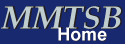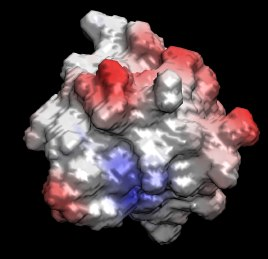MMTSB Tool Set - Continuum electrostatics calculationsObjective and Overview

In this lab we will learn how to run and use continuum dielectric electrostatic calculations using CHARMM through the MMTSB Tool Set.### 1. Calculation of electrostatic solvation energies

We will use the engrailed homeodomain again. The structure is available from the Protein Data Bank with the PDB code 1ENH. First we need to obtain a structure that has all of the atoms present (including hydrogens) and center the molecule with respect to the origin:
```    convpdb.pl -nsel protein 1ENH.pdb > 1enh.protein.pdb
complete.pl 1enh.protein.pdb > 1enh.allh.pdb
convpdb.pl -center 1enh.allh.pdb > 1enh.center.pdb
```
Electrostatic solvation energies according to Poisson theory can be obtained using the CHARMM PBEQ module with the following command:
```    pbCHARMM.pl 1enh.center.pdb
```
The Poisson equation is solved on a grid where the grid resolution determines the accuracy of the result. You can change the grid resolution with the parameter 'dcel':
```    pbCHARMM.pl -par dcel=0.5 1enh.center.pdb
```
Try using values from 0.5 (default) to 0.2. How does the energy change?

The GBMV generalized Born approximation can also be used to calculate electrostatic solvation energies. Try calculating the GB energy for the same system with the following command:
```    enerCHARMM.pl -par gb,nocut -out gb 1enh.center.pdb
```
How much does the result deviate?

An alternative to the commonly employed molecular surface definition (that is used by default) a smoother surface based on overlapping van der Waals spheres is sometimes used. This surface is turned on with the 'smooth' parameter:
```    pbCHARMM.pl -par dcel=0.5,smooth 1enh.center.pdb
```
The GBSW method matches the smooth surface. GBSW energies are calculated with:
```    enerCHARMM.pl -par gb=gbsw,nocut -out gb 1enh.center.pdb
```
Compare all of the results obtained so far.

### 2. Calculation of electrostatic maps by solving the Poisson equation

Maps of electrostatic potentials around biomolecules are useful in determining possible interaction sites for protein-protein and protein-ligand interactions. Electrostatic potentials can be obtained by solving the Poisson equation for a given molecular structure. In this exercise we will use CHARMM to carry out such a calculation and then use VMD in order to visualize the results.

We will use the tool pbCHARMM.pl again in order to calculate the electrostatic potential on a grid:
```    pbCHARMM.pl -emap phi.dx 1enh.center.pdb
```
The electrostatic potential is best visualized by projection onto the molecular surface. The molecular surface can be obtained from the distribution of the dielectric constant with:
```    pbCHARMM.pl -dx -grid epsx grid.dx 1enh.center.pdb
```
Now use VMD and load the molecule, the molecular surface, and the electrostatic potential. Color the molecular surface according to the potential and compare with the location of the charged residues on the surface.

Calculate the electrostatic map also for the smooth van der Waals-based surface and compare. Because the surface has changed, both the electrostatic potential and surface need to be recalculated.

You can change the dielectric constant and introduce a salt concentration of the solute. The corresponding parameters are epsw (external dielectric constant) and pbionconc (ion concentration). For example, epsilon=50 and an ion concentration of 0.1 M is modeled with:
```    pbCHARMM.pl -emap modphi.dx -par epsw=50,pbionconc=0.1 1enh.center.pdb
```
Compare the resulting map with the result obtained previously.

Written by M. Feig